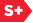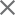### Proceedings Paper

Optical localization: using fixed point placement to simplify the invariant measure
Author(s): Glen J. Kissel
Format Member Price Non-Member Price
PDF \$17.00 \$21.00

Paper Abstract

Optical localization in one-dimensional very long disordered photonic bandgap structures is characterized by the upper Lyapunov exponent of the infinite random matrix product model. This upper (and positive) Lyapunov exponent, or localization factor (inverse localization length), is, at least theoretically, calculable from Furstenberg's formula. This formula not only requires the probability density function for the random variables of the random transfer matrix, but also requires the invariant probability measure of the direction of the vector propagated by the long chain of random matrices. This invariant measure is generally only calculable numerically, and when the transfer matrices are in the usual real basis, or even a plane wave basis, the invariant measure takes no consistent form. However, by using a similarity transformation which moves the fixed points of the bilinear transformation corresponding to the long random matrix product, we can put the random matrix product in the so-called real hyperbolic-canonical basis. The aim of this change of basis is to place the attracting fixed point at the origin and the repelling fixed point at infinity. The invariant measure, as a result, is dramatically simplified, approaching a probability mass function with mass concentrated around the angle zero.

Paper Details

Date Published: 4 September 2008
PDF: 12 pages
Proc. SPIE 7029, Metamaterials: Fundamentals and Applications, 70290K (4 September 2008); doi: 10.1117/12.797333
Show Author Affiliations
Glen J. Kissel, Univ. of Southern Indiana (United States)

Published in SPIE Proceedings Vol. 7029:
Metamaterials: Fundamentals and Applications
Mikhail A. Noginov; Nikolay I. Zheludev; Allan D. Boardman; Nader Engheta, Editor(s)